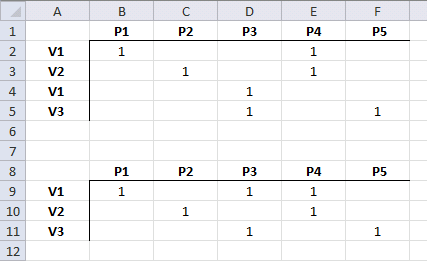Author: Oscar Cronquist Article last updated on November 30, 2012

Oscar,

I'm hoping you can help. I am trying to group a number of rows together by the first column, providing a union of the column values. In the example below, we are looking to Vendors V1-V3 to sell us some subset of products P1-P5.

; P1 ; P2 ; P3 ; P4 ; P5
V1 ; 1 ; ; ; 1 ;
V2 ; ; 1 ; ; 1 ;
V1 ; ; ; 1 ; ;
V3 ; ; ; 1 ; ; 1

Once transformed, I would like to see the following:

; P1 ; P2 ; P3 ; P4 ; P5
V1 ; 1 ; ; 1 ; 1 ;
V2 ; ; 1 ; ; 1 ;
V3 ; ; ; 1 ; ; 1Extract unique distinct values

Array formula in cell A9:

=INDEX(\$A\$2:\$A\$5,MATCH(0,COUNTIF(\$A\$8:A8,\$A\$2:\$A\$5),0))

Copy and paste array formula in A9 down as far as needed.

Read this post for more details: How to extract a unique distinct list from a column

Group values

Array formula in cell B9:

=IF(SUMPRODUCT((\$A9=\$A\$2:\$A\$5)*(B\$8=\$B\$1:\$F\$1)*(\$B\$2:\$F\$5=1))>0,1,"")

How to create an array formula

1. Select cell B9
2. Click in formula bar3. Copy and paste above array formula
4. Press and hold Ctrl + Shift
5. Press Enter

How to copy array formula

1. Select cell B9
2. Copy cell (Ctrl + c)
3. Select cell range C9:F9
4. Paste (Ctrl + v)
5. Select cell range B9:F9
6. Copy cell range (Ctrl + c)
7. Select cell range B10:F11
8. Paste (Ctrl + v)

### Explaining array formula in cell B9

Step 1 - Find matching vendor

\$A9=\$A\$2:\$A\$5

becomes

"V1 "={"V1 ";"V2 ";"V1 ";"V3 "}

and returns

{TRUE;FALSE;TRUE;FALSE}

Step 2 - Find matching product

B\$8=\$B\$1:\$F\$1

becomes

" P1 "={" P1 "," P2 "," P3 "," P4 "," P5"}

and returns

{TRUE, FALSE, FALSE, FALSE, FALSE}

Step 3 - Find values equal to 1

\$B\$2:\$F\$5=1

becomes

{1," "," ",1," ";" ",1," ",1,"";" "," ",1," "," ";" "," ",1," ",1}=1

and returns

{TRUE, FALSE, FALSE, TRUE, FALSE;FALSE, TRUE, FALSE, TRUE, FALSE;FALSE, FALSE, TRUE, FALSE, FALSE;FALSE, FALSE, TRUE, FALSE, TRUE}

Step 4 - Return array of values

(\$A9=\$A\$2:\$A\$5)*(B\$8=\$B\$1:\$F\$1)*(\$B\$2:\$F\$5=1)

becomes

({TRUE;FALSE;TRUE;FALSE})*({TRUE, FALSE, FALSE, FALSE, FALSE})*{TRUE, FALSE, FALSE, TRUE, FALSE;FALSE, TRUE, FALSE, TRUE, FALSE;FALSE, FALSE, TRUE, FALSE, FALSE;FALSE, FALSE, TRUE, FALSE, TRUE})

and returns

{1, 0, 0, 0, 0;0, 0, 0, 0, 0;0, 0, 0, 0, 0;0, 0, 0, 0, 0}

Step 5 - Sum array of values

SUMPRODUCT({1, 0, 0, 0, 0;0, 0, 0, 0, 0;0, 0, 0, 0, 0;0, 0, 0, 0, 0})

returns 1 in cell B9.